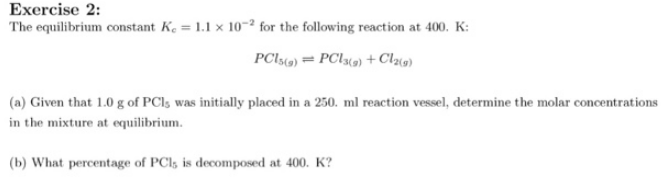# Problem: The equilibrium constant Kc = 1.1 x 10-2 for the following reaction at 400 K: PCl5 (g) ⇌ PCl3 (g) + Cl2 (g) (a) Given that 1.0 g of PCl5 was initially placed in a 250 ml reaction vessel, determine the molar concentrations in the mixture at equilibrium. (b) What percentage of PCl5 is decomposed at 400 K?

###### FREE Expert Solution
93% (58 ratings)###### Problem Details

The equilibrium constant Kc = 1.1 x 10-2 for the following reaction at 400 K:

PCl5 (g) ⇌ PCl3 (g) + Cl2 (g)

(a) Given that 1.0 g of PCl5 was initially placed in a 250 ml reaction vessel, determine the molar concentrations in the mixture at equilibrium.

(b) What percentage of PCl5 is decomposed at 400 K?